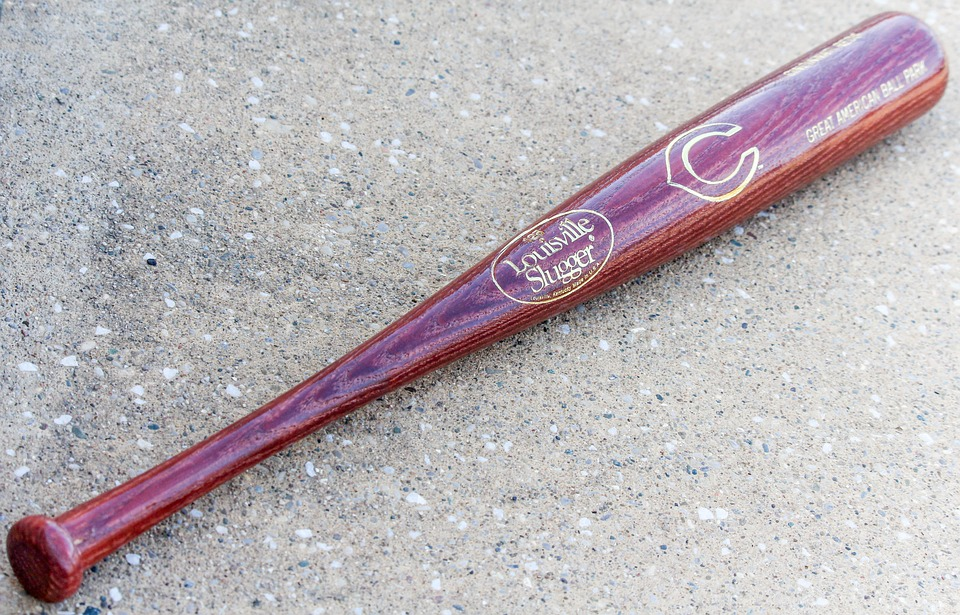# Young’s modulus or longitudinal modulus of elasticityYoung’s modulus is a parameter that characterizes the behavior of an elastic material, depending on the direction in which a force is applied. It is one of the most widely used methods for determining the elasticity of a material.

For a linear and isotropic elastic material, Young’s modulus has the same value for tension as for compression, being a constant independent of stress as long as it does not exceed a maximum value called the elastic limit, and is always greater than zero: if a bar is tractioned, it increases in length.

Both Young’s modulus and yield strength are different for different materials. The modulus of elasticity is an elastic constant which, like the yield strength, can be found empirically by tensile testing of the material. In addition to this longitudinal modulus of elasticity, the transverse modulus of elasticity of a material (shear modulus) can be defined.

## Who does Young’s module refer to?

To understand how tensile testing works and to take full advantage of the possibilities of the universal machine, we must know each and every one of the methods to be used to obtain our objectives in an optimal way. In this case, Young’s modulus refers to the scientist Thomas Young, who studied this concept during the 19th century. However, the method was developed until 1727 by Leonhard Euler and was not put into practice in its current form until 1782 when Giordani Riccati performed the first experiments.

Young’s modulus, also called longitudinal elasticity, is a parameter that manages to reveal the behavior of an elastic material as a function of the type of force applied to it and the consequent increase or decrease in the length of that material. Therefore, what it seeks is to obtain the relationship between the stress applied to the object in its longitudinal axis and the deformation measured in that same axis. Thus, it measures its elastic behavior and also predicts the stretching of a given material.For example, if we apply the same stress on a shower sponge and a baseball bat, we can see that the elastic deformation is much smaller in the bat than in the sponge. This means that Young’s modulus will be higher in the bat than in the rubber and that it will be necessary to apply a higher stress on it to get the same deformation.## Formula

The way to calculate Young’s modulus is as follows:

E = σ/ϵ

Being:

•     E = Young’s modulus, in pascal.

• σ = uniaxial stress or uniaxial force per unit area, in pascal.

• ε = deformation or proportional deformation (this means the change in length divided by the original length).

## Aplications

Young’s modulus is applicable for a variety of functions, such as on a construction site when a rock is the support for other structures (the foundation), to compare the performance of different materials or to measure the stiffness of a solid material.

In short, Young’s modulus is vital in determining the tensile strength of a material or object. If you want to know more about this or other similar topics do not hesitate to take a look at the Servosis blog. In addition, in our store you will find the machinery that best suits your needs and those of your team.